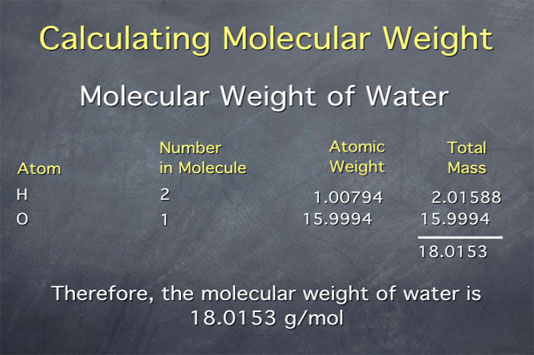# Stoichiometry Tutorials: Using Molecular Weight

(from a complete OLI stoichiometry course)

A previous tutorial shows how to calculate the molecular weight of a substance from the atomic weights given. On this page, we use the molecular weight to convert between the macroscopic scale (grams of a substance) and the microscopic scale (number of molecules of that substance).

### Using Molecular Weight Movie Text

Molecular weight is an important tool in stoichiometry, because it allows us to connect between the macroscopic scale, which is what we percieve in our everyday lives, to the molecular scale, where chemistry occurs. On the macroscopic scale, we think in terms of grams of a substance, while on the molecular scale, we think in terms of numbers of molecules, in moles.

To connect between the macroscopic and molecular scale, we use the molecular weight. For example, the molecular weight of water is 18.015 grams per mole. This gives us the two ratios shown in the image below, which we can use to convert between grams and moles.We can use this to get from a quantity expressed in grams like 7.4 grams of water, a macroscopic quantity that we might weigh out in a lab, to the quantity expressed in number of moles of water, a molecular quantity. To do this, we take our existing 7.4 grams water and multiply by our ratio of 1 mole of water per 18.015 grams of water. Remember, this ratio is equal to one, so multiplying by it converts the units without making any difference to the actual quantity of water. Next, we do the math and get a value 0.41. The units of grams of water cancel, so the units of the result are moles of water.